## 14.12. Rotating graphs

The library supports an arbitrary rotation of a the plot area as well as some special convenience method to rotate the plot area 90 degree which most often is used to draw a horizontal bar graph instead of a (standard) vertical bar graph.

### Caution

Adding a rotation transformation will make the graph generation slightly slower since each point of the graph as to go through a transformation step before being stroked on to the image. The library tries to mitigate this as much as possible by using a pre-calculated transformation matrix and also makes further optimizations for the special case of 90 degree rotations.

### Caution

Any background images (see Section 14.15) will not be rotated with the graph. This limitation exists since the performance and memory usage of doing real image transformation in PHP would be too poor. Any background images needing rotation must be rotated outside in some image manipulation program (e.g. Gimp, IrfanView).

When a plot area is rotated there are two things to be aware of

1. individual labels on the axis are not rotated

The design decision behind this is that bit mapped fonts cannot be arbitrarily rotated and rotating TTF fonts will decrease (in general) the readability. If angle rotation is needed on the labels it is still possible to use the method `Axis::SetLabelAngle()`

### Note

Since the anchor point for labels is by default the optimum for graph at 0 degree the anchor point and alignment for the labels on the axis should probably be adjusted to get a better visual appearance on the rotated graph. This is accomplished by the method `Axis::SetLabelAlign()`

2. any background image or background gradient is not rotated

The design decision behind this is purely computational Doing a full image rotation would be excruciating CPU intensive using PHP.

### 14.12.1. Free rotation of the plot area

The rotation of the plot area is controlled with the following two methods

• `Image::SetAngle(\$aAngle)`

"`\$Angle`" = Angle of rotation specified in degrees. A positive angle specifies a clockwise rotation.

• `Image::SetCenter(\$aX, \$aY)`

Specifies the center of rotation

The Image class is the lowest graphic layer in the library and it is access through the instance variable "`\$img`" of the Graph class. So for example to rotate a plot are 45 degree the following line has to be added

 ```1 ``` `\$graph->img->SetAngle(45);`

By default the center of rotation will be the center point of the plot area. The following examples will clarify this. We will use the (very) basic graph shown in Figure 14.74 to demonstrate rotation.

Figure 14.74. Original unrotated graph `(rotex0.php)`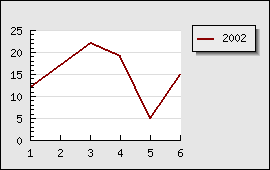In Figure 14.75 and Figure 14.76 we have rotated the plot area around (the default) the center of the plot area

 Figure 14.75. Rotating the plot area 45 degrees `(rotex1.php)`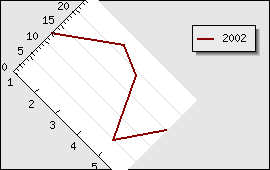Figure 14.76. Rotating the plot area 90 degrees `(rotex2.php)`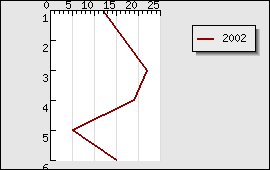In the above two example the center of the rotation was the center point of the plot area. If we instead change the center of rotation to be the center of the entire graph we get the result shown in Figure 14.77 and Figure 14.78 .

 Figure 14.77. Rotating the plot area 45 degrees `(rotex3.php)`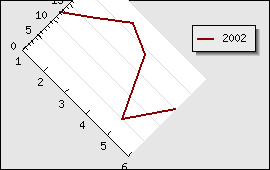Figure 14.78. Rotating the plot area 90 degrees `(rotex4.php)`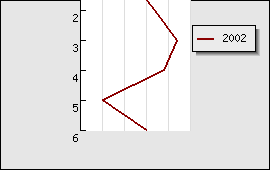As a final example in Figure 14.79 we show the result of rotating the plot area -30 degree around the bottom left point in the graph

Figure 14.79. Rotating the plot area -30 degree around the bottom left corner `(rotex5.php)`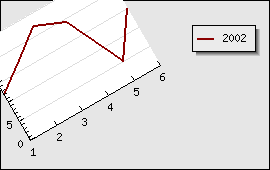### 14.12.2. Rotating the plot area 90 degree

As can be seen above in Figure 14.76 and Figure 14.78 the rotation does not alter the overall size and margin of the graph even though we probably should do so in order to better accommodate the rotated plot areas topography. It is of course perfectly possible to adjust the size of the graph manually.

The slight complication with general rotation is that the margins also rotates, this means that if the graph is rotated 90 degrees the left margin in the image was originally the bottom margin. In additional by default the center of the rotation is the center of the plot area and not the entire image (if all the margins are symmetrical then they will of course coincide). This means the center of the rotation will move with the margin (since the specify the exact location of the plot area)..

So for the case of rotating a graph 90 degree the library provides a convenience method to do both the rotation and specifying the margin at the same time (to avoid the mental exercise described above) by providing the method

• ```Set90AndMargin((\$aLeft=0,\$aRight=0,\$aTop=0,\$aBottom=0) ```

Rotates the plot area 90 degrees and sets the graph margin areas

This method is probably most commonly used with bar graphs to create horizontal instead of vertical bars. See the section on bar graphs, ??, for more example on this.